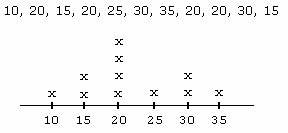Definition Of Mode

Mode is a number that occurs most frequently in the data set.

The data set with more than one mode is called Multimodal.

Examples of Modeideo Examples: Mean Median and Mode

In the given line plot, most number of cross (4) are shown against 20. So 20 is the mode of the given line plot.
The mode of the set M, S, R, S, S, M, M, R, M, R is M, as M is occurred more frequently than S and R.
60, 55, 59, 56, 61, 62, 62, 62, 57, 61
60 in the data set occur only once.
55 in the data set occur only once.
59 in the data set occur only once.
56 in the data set occur only once.
61 in the data set occur twice.
62 in the data set occur thrice.
57 in the data set occur only once.
So, the mode for the above data set is 62 as it occurred most frequently.

A. 11
B. 7
C. 16
D. 14